# Test: Jugs And Mugs - 2

## 20 Questions MCQ Test Online MCQ Tests for Class 2 | Test: Jugs And Mugs - 2

Description
Attempt Test: Jugs And Mugs - 2 | 20 questions in 40 minutes | Mock test for Class 2 preparation | Free important questions MCQ to study Online MCQ Tests for Class 2 for Class 2 Exam | Download free PDF with solutions
QUESTION: 1

### The object that can be measured in kg is ______.

Solution:

Kilogram is used to. Measure weight of heavy objects. A bag of vegetable is the heavy so it is measured in kg.

QUESTION: 2

### Which is the smallest?

Solution:

10 metre is the smallest length.

QUESTION: 3

### What should be the weight on the other side of a balance so that it goes down? If one side has weight of 1 kg.

Solution:

Pan which will have heavy object will go down. 2 kg is the heaviest.

QUESTION: 4

Rahul had a bucket full of 20l water. It is telling about its ______.

Solution:

Bucket having 20 I water is telling about its capacity.

QUESTION: 5

Which of the following can be used to roughly measure the length of a book?

Solution:

All of these objects can be used roughly to measure length of a book.

QUESTION: 6

How can you measure length of your class room accurately and faster?

Solution:

You can measure length from all of the these. But, metre tape can measure length accurately and fastly.

QUESTION: 7

Which is same as 6 m?

Solution:

1m =100 cm
6m = 6 × 100 cm

QUESTION: 8

Which is the most appropriate object to measure the weight of a water melon?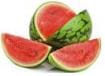Solution:

Balance is used to measure weight of water melon. Rest are used to measure lengths.

QUESTION: 9

If the weight of 6 oranges is equal to 4 apples. Then weight of 12 oranges will be equal to

Solution:

Weight of 6 oranges = weight of 4 apples.
Weight of 6 × 2 = 12 oranges = weight of 4 × 2 = 8 apples.

QUESTION: 10

Fill in the blank Weight of 300 g of oranges is ______ than 50 g of grapes.

Solution:

Weight of 300 g of oranges is greater than 50 g of grapes.

QUESTION: 11

Write the following in ascending order:
2 m, 300 cm, 100 cm

Solution:

100 cm < 2 m < 300 cm
or 1m
or 3m

QUESTION: 12

Arrange the following objects in the order of their length from smallest to longest.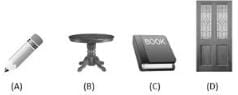Solution:

Length of pencil < length of book < length of table < length of door. So, A < C < B < D.

QUESTION: 13

Approximately how many pencils will measure the length of book as shown in the figure?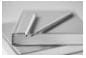Solution:

From the image, it can be observed that 2 pencils are required to measure a book.

QUESTION: 14

How many millilitres are there in 2 litres?

Solution:

Ans: c

Explanation: The prefix “milli” means one thousand, that means there are 1000 millilitres in one litre. Therefore two litres will contain 2000 millilitres.

QUESTION: 15

Each ant drinks 1 ml of kheer. So 1000 ants drink ________ml.

Solution:

1000 x 1ml = 1000 ml

QUESTION: 16

Choose the odd one out

Solution:

Only option has discussed about weight. Rest have the measurement of length.

QUESTION: 17

Three glasses of water can fill a water-bottle. How many glasses of water are required to fill 4 such water-bottles?

Solution:

3 glasses of water can fill a water - bottle.
3×4=12glasses of water can fill 4 water-bottles.

QUESTION: 18

Match the best estimate of measurement of weight with the objects given.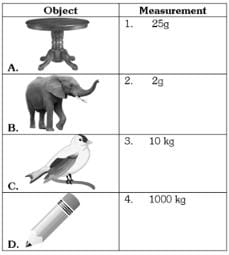Solution: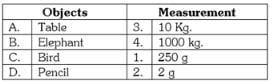QUESTION: 19

Read the statements and mark them as True (T) or False (F). Gram is a unit to measure weight of a lion. Kilogram is a unit to measure weight of a sack of rice.

Solution:

Statement A is wrong. Gram is a unit to measure small things not a big lion.

QUESTION: 20

How many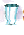small glasses will fill half the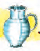jug?

Solution:Use Code STAYHOME200 and get INR 200 additional OFF Use Coupon Code